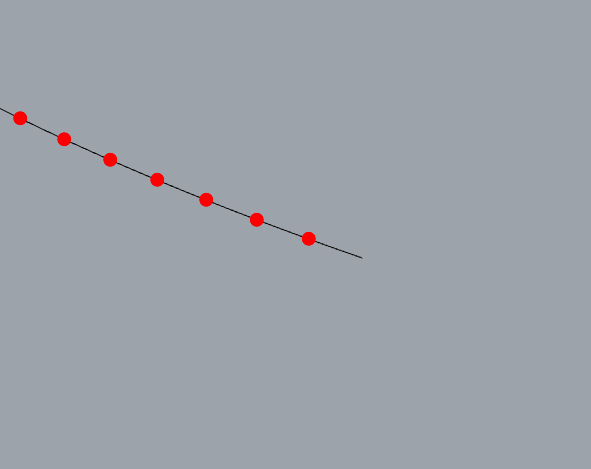# 《RhinoPython 101》中关于R1与R3点的转换，为什么曲线端点无法显示点？import rhinoscriptsyntax as rs

def main():
curve_id = rs.GetObject(“Select a curve to sample”, 4, True, True)
if not curve_id: return
rs.EnableRedraw(False)
t = 0
while t <= 1.0:
t += 0.002
rs.EnableRedraw(True)

domain = rs.CurveDomain(curve_id)
r1_param = domain + parameter * (domain - domain)
r3point = rs.EvaluateCurve(curve_id, r1_param)
if r3point:
rs.ObjectColor(point_id, parametercolor(parameter))

def parametercolor(parameter):
red = 255 * parameter
if red<0: red=0
if red>255: red=255
return (red, 0, 255-red)

if name==“main”:
main()

``````t = 0
while t <= 1.0:
print t
t += 0.002
``````

2 个赞

3 个赞

1 个赞